Back

## Criss Cross Triangles

We can think of an angle as the measure of a turning motion or rotation.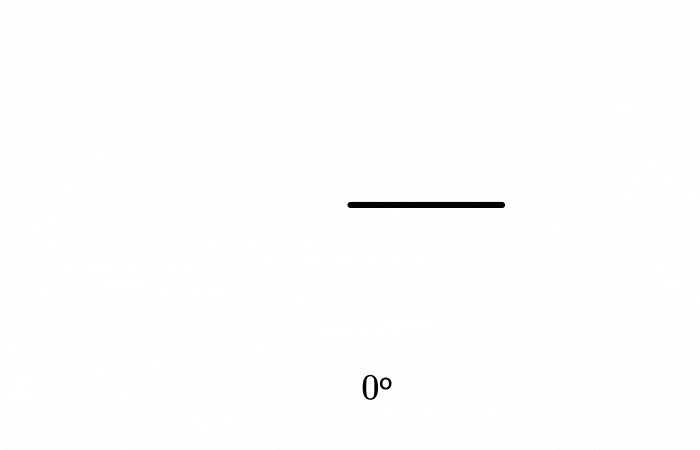When multiple angles are in the same diagram, they can be related to one another in several ways. We can use these relationships as tools for solving angle hunt problems. Keep reading to see some of these tools used, or jump ahead to today's challenge if you're ready.

Here are some ways we can relate angles:

1. When angles combine to form a straight line, their measures add to $180^\circ.$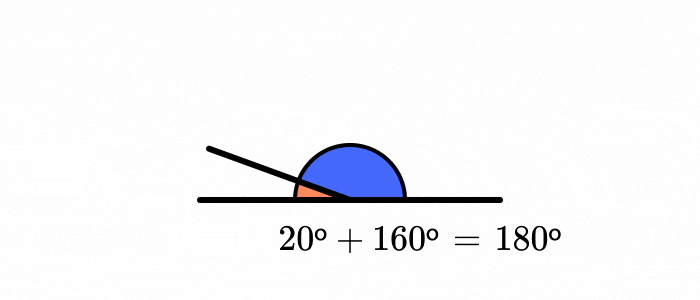2. When two lines intersect, opposite angles have equal measures.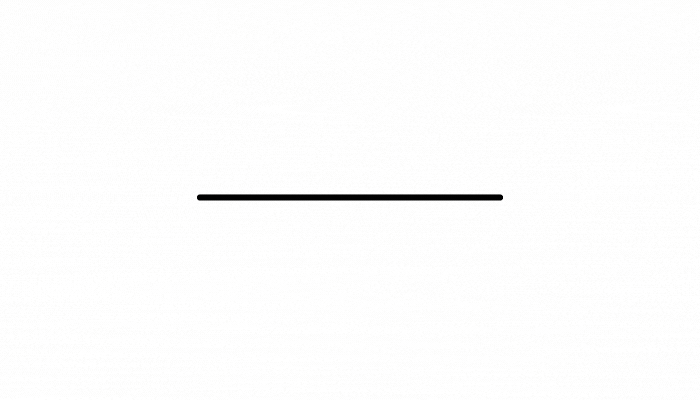3. The measures of a triangle's interior angles add to $180^\circ.$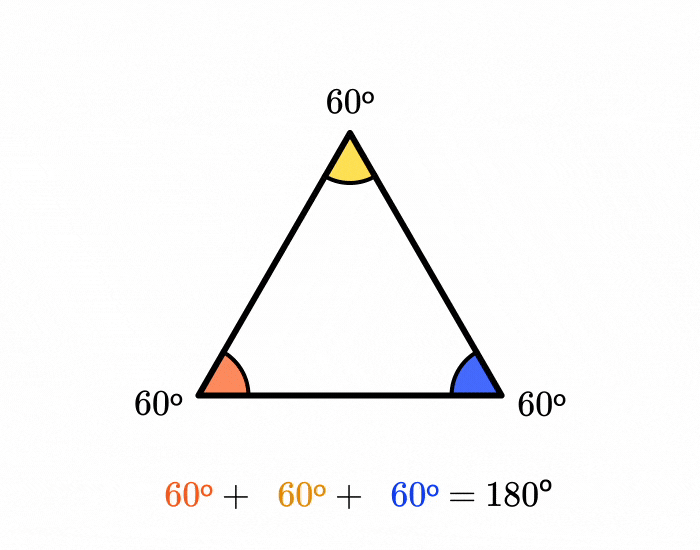Let's see some ways we can use these relationships. We'll start by finding the measure of the missing angle below.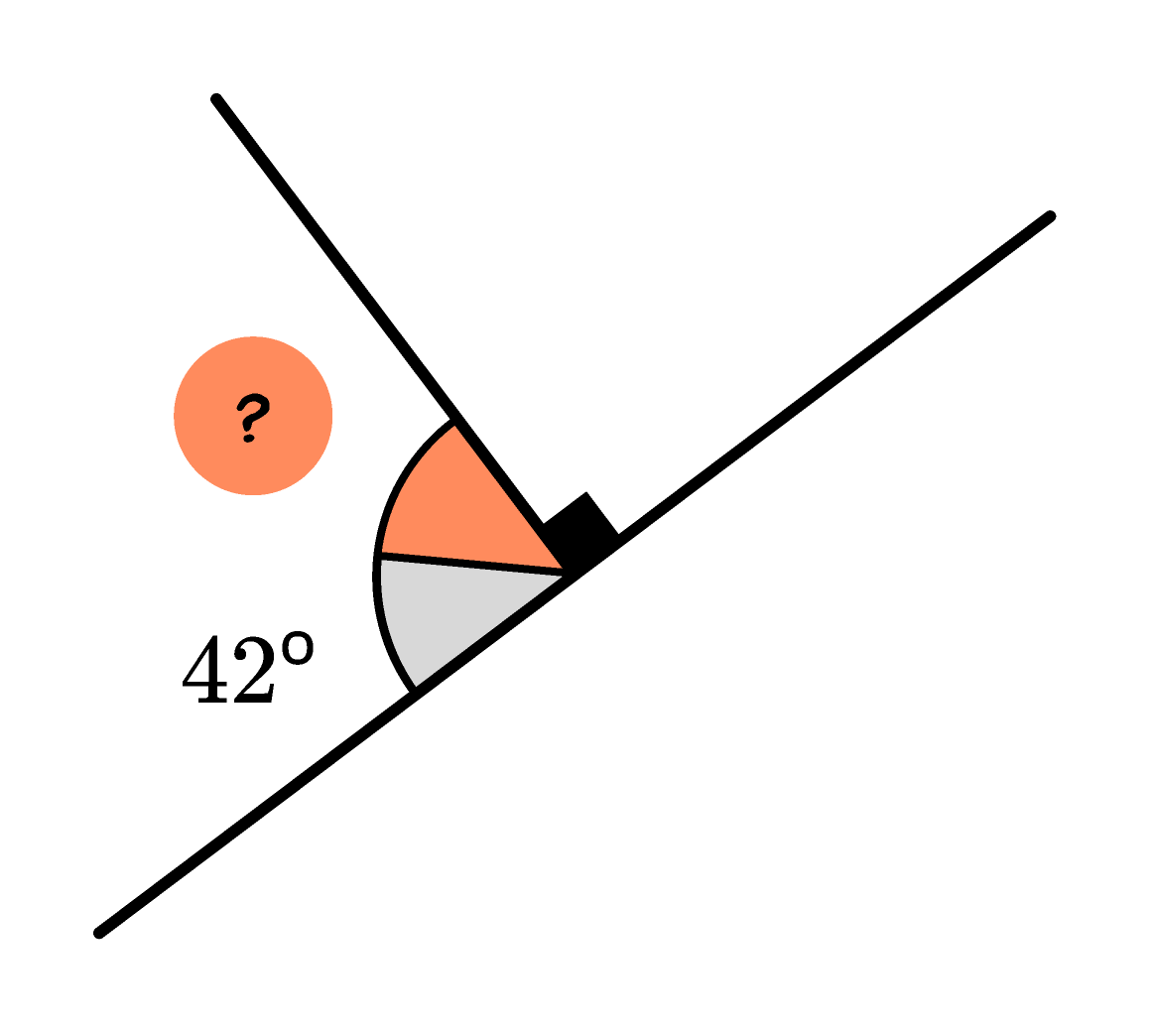We see three angles that combine to form a straight line. By Fact $1,$ we know that their measures add to $180^\circ.$ Additionally, the black square indicates a right angle, which has a measure of $90^\circ.$ So, we know that $42\ +\ ? + 90 = 180.$ Adding $42$ and $90$ gives us $132,$ so the missing angle has measure $132^\circ$ less than $180^\circ.$ Subtracting $132^\circ$ from $180^\circ$ gives us $48^\circ.$

The next problem is a bit more complex. How can we find the value of $x?$There are two triangles in the figure, so maybe Fact $3$ will be helpful. Applying this fact to the triangle on the left won't tell us much, but applying it to the triangle on the right will let us find the measure of its unmarked angle. The measures of all three angles of the triangle add to $180^\circ,$ with the two marked angles' measures adding to $60^\circ + 80^\circ = 140^\circ.$ This means the unmarked angle must have a measure of $180^\circ - 140^\circ = 40^\circ.$

Fact $2$ tells us that the left triangle's unmarked angle also has a measure of $40^\circ.$ Now that we know this, Fact $3$ should be more helpful. We can relate the measures of all three angles of the triangle by the sum $x + x + 40 = 180.$ This means $x + x$ is equal to $180 - 40 = 140,$ so $x = 140 \div 2 = 70.$

See if you can use these angle relationships to tackle the angle hunt challenge below.

# Today's Challenge

An isosceles triangle is a triangle with two sides that are the same length. Isosceles triangles also have two angles with the same measure — the angles opposite the equal sides.

In the image below, all the orange segments are the same length. What is the measure of the blue angle?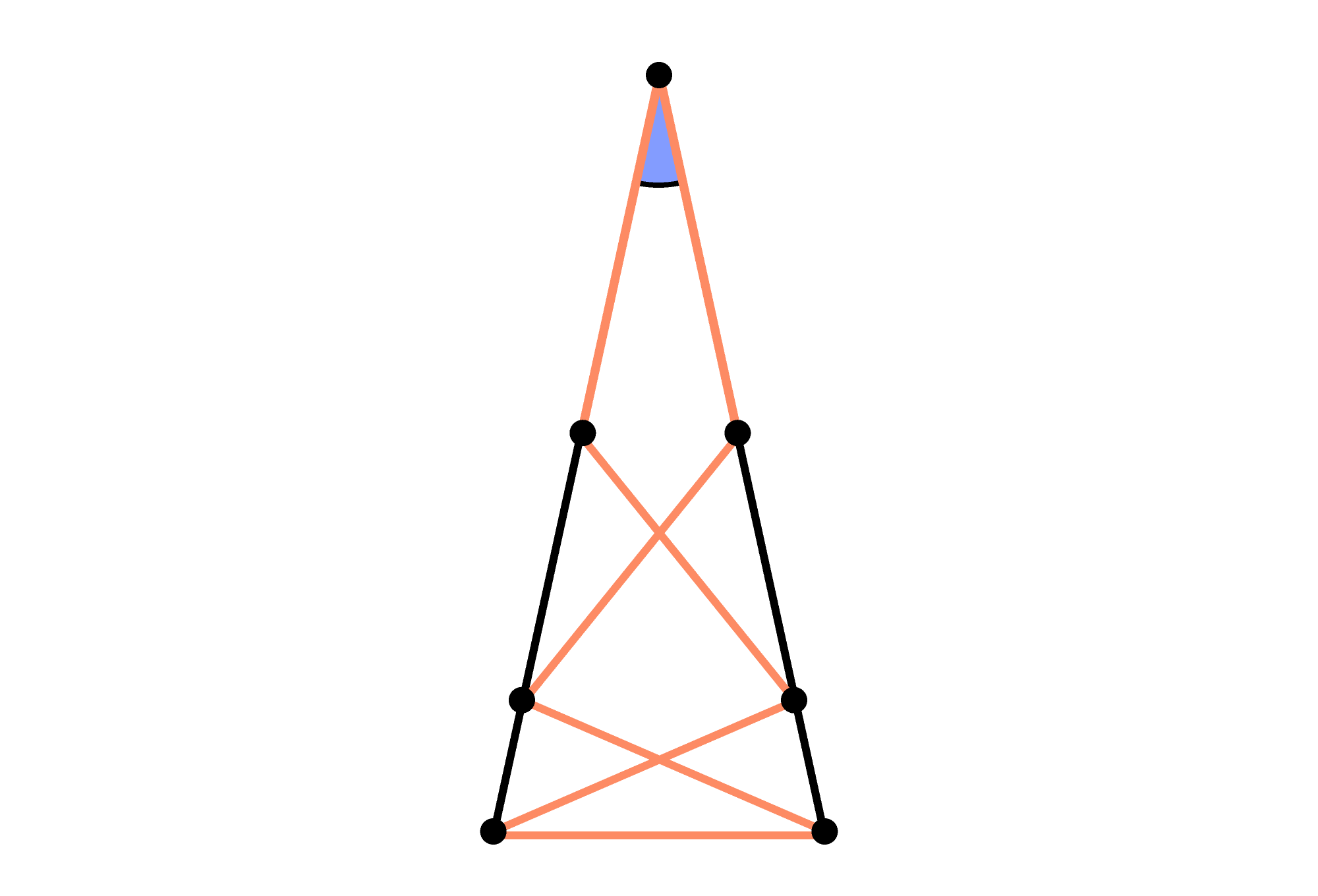Hint: If you know two angles of a triangle, what can you say about the third?We are sunsetting our community features by July 2, 2021. Learn more here.
×

Problem Loading...

Note Loading...

Set Loading...